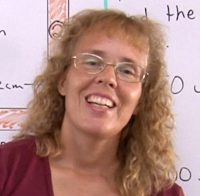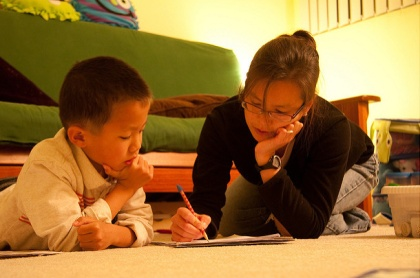^
You are here: HomeLessons → Word problemsHello!
In this article, I want to talk about MATH WORD PROBLEMS. They indeed give many children "problems", but that doesn't have to be!Image by RadioFlyer007 (black border removed)

Here's the outline of the article:

## The problem

Have you ever noticed this kind of "recipe" for math lessons in many math books?

LESSON X

Explanation and examples.

Numerical exercises.

A few word problems.

In other words, the word problems are usually in the END of the lesson, and just a few. But worse... if the lesson is about topic X, then the word problems are usually about the topic X too!

Children might be learning about multi-digit multiplication, or subtraction, or dividing decimals. After the calculation exercises come some word problems, which oddly enough are solved by using the exact operation just practiced!

Worse YET... typically the word problems in elementary grades only have TWO numbers in them. So, even if you didn't understand a word in the problem, you might be able to do it. (The misguide to problem solving at the end of this article refers to this also.)

Just try: the following made-up problem is in FINNISH... and let's say it is found within a long division lesson. I assume now that you do NOT know Finnish — but can you solve it?

Kaupan hyllyillä on 873 lakanaa, 9:ää eri väriä. Joka väriä on saman verran. Kuinka monta lakanaa on kussakin värissä?

Drag your mouse over the white space below to see the translation (highlight it).

The store has 873 sheets in 9 different colors. There is the same amount of sheets for each color. How many sheets of each color are there?

My thought is that over the years, when children are exposed to such lessons over and over again, they figure out that it's mentally less demanding to NOT even READ the problem too carefully! Why bother? Just take the two numbers, divide (or multiply, or add, or subtract) them, and that's it. They "learn" (intelligently) this unspoken rule:

"Word problems in math books are solved by some routine or rule that you find in the beginning of that particular lesson."

It is no wonder that our students are not able to solve anything that's different from that routine!

## The solution

How can you avoid this terrible situation?

Children simply need to be given good problems to solve. That way, they will get used to THINKING.

These good word problems should advance in difficulty over the grades, and foster children's logical thinking. ONE-step problems are adequate for 1st and 2nd grades, and then here and there mixed in with other types of problems.

In early elementary grades, one needs to mix up the (often one-step) word problems so that not all of them are solved by the operation just studied. I have done that often in my Math Mammoth books.

But children need to start solving MULTI-step problems as soon as they can, including even in 1st grade. (And yes, Math Mammoth books do include some two-step problems starting in 1st grade materials.)

For more word problems than in your current math curriculum, check the list of problem solving resources at the end of this article.

What about Math Mammoth and word problems? you might ask. I don't currently have a single book with only word problems (though we're hoping to make one, one day). Instead, word problems are simply present in ALL of the Math Mammoth materials.

Here's how to help a student with problem solving and word problems using Math Mammoth. Such a student could go through all of the Math Mammoth Light Blue or Blue series, starting from grade 1 materials, and do ONLY the word problems and related lessons.

## Problems to ponder

Next, let's take a look at some PROBLEMS.First, look at this example problem from a Russian fourth grade book:

An ancient artist drew scenes of hunting on the walls of a cave, including 43 figures of animals and people. There were 17 more figures of animals than people. How many figures of people did the artist draw?

A similar problem is included in the 5th grade Singapore textbook:

Raju and Samy shared \$410 between them. Raju received \$100 more than Samy. How much money did Samy receive?

Now, these are not anything spectacular. You can solve these types of problems for example by using a bar model (image from Math Mammoth grade 5 Light Blue series):Or, you can solve them with arithmetic: simply take away the given difference from the total, then divide the remaining amount evenly, and lastly add the given difference to one person's part.

Yet in the U.S., these kind of problems are generally introduced in Algebra 1 — in ninth grade(!), AND they are only solved using algebraic means.

Here is another example, of which I remember feeling aghast, found in a modern U.S. algebra textbook:

Find two consecutive numbers whose product is 42.

Third-grade children should know multiplication well enough to quickly find that 6 and 7 fit the problem! Why use a "backhoe" (algebra) for a problem you can solve using a "small spade" (simple multiplication)!

I know some will argue and say, "Its purpose is to learn to set up an equation." But for that purpose I would use a bigger number and not 42. (Try 3422.) Don't such simple problems in algebra books just encourage students to forget common sense and simple arithmetic?

Another example, a 3rd grade problem from Russia:

A boy and a girl collected 24 nuts. The boy collected two times as many nuts as the girl. How many did each collect?

You can draw a boy and a girl, draw two pockets for the boy, and one pocket for the girl. This visual representation easily solves the problem.

Here is an example of a Russian problem for grades 6-8:

An ancient problem. A flying goose met a flock of geese in the air and said: "Hello, hundred geese!" The leader of the flock answered to him: "There is not a hundred of us. If there were as many of us as there are and as many more and half many more and quarter as many more and you, goose, also flied with us, then there would be hundred of us." How many geese were there in the flock?

(You can solve this in many different ways — and that is a often the case withe good word problems.)

So, once again, the only true way of learning to solve word problems is .... TO SOLVE LOTS OF THEM. They don't have to be real-life or involve awkward numbers (such as occur in real life). Realistic, complex problems might be good for a "spice", but not for the "main course". "Fantastic" (unreal) problems are fine.

One of my girls used to cherish the word problems in my Math Mammoth books MORE than the other kinds of problems. And the general consensus of the feedback I hear from Math Mammoth users is that once children get used to solving a variety of word problems, it's no longer a problem!

Some children, if not used to solving word problems, may need to start from the beginning — with a collection of simple one-step word problems (mixed up). But that's okay. They will learn!

Lastly, a joke by Lynn Nordstrom:

## Student's Misguide to Problem Solving

Rule 1: If at all possible, avoid reading the problem. Reading the problem only consumes time and causes confusion.

Rule 2: Extract the numbers from the problem in the order they appear. Be on the watch for numbers written in words.

Rule 3: If rule 2 yields three or more numbers, the best bet is adding them together.

Rule 4: If there are only 2 numbers which are approximately the same size, then subtraction should give the best results.

Rule 5: If there are only two numbers and one is much smaller than the other, then divide if it goes evenly -- otherwise multiply.

Rule 6: If the problem seems like it calls for a formula, pick a formula that has enough letters to use all the numbers given in the problem.

Rule 7: If the rules 1-6 don't seem to work, make one last desperate attempt. Take the set of numbers found by rule 2 and perform about two pages of random operations using these numbers. You should circle about five or six answers on each page just in case one of them happens to be the answer. You might get some partial credit for trying hard.

I hope your students do not fit the above joke.

## Sources and further resources

Math Stars
Free newsletters with the purpose of challenging students with good problems that inspire curiosity. Math Stars are in sets for Grades 1-8 and include commentaries for teachers.

Problem Solving Decks
A free collection of problems for grades 1-8, originally published by the North Carolina State Board of Education.Math Puzzles and Brainteasers, Grades 6-8
A book with over 300 delightful puzzles and problems that teach math and problem solving.

Ray's Arithmetic
Ray's Arithmetic is an old math book, full of word problems, starting from the simplest. It basically uses word problems to teach arithmetic. It is available FREE online.

3rd Grade Math Word Problems Worksheets
Look especially for the MIXED word problem worksheets in this collection. The site offers word problem worksheets for all of the grades 1-5 (look in the menu).

Word Problems in Russia and America
An article by Andrei Toom. Contains some good example problems from Russia!Step-by-Step Problem Solving, Grade 4
A problem-solving workbook that provides step-by-step instruction, problem pages at varied levels of difficulty, a math strategies overview, and a complete answer key. The book utilizes bar models just like Math Mammoth and Singapore Math. Other grades available also.

Mixed operations word problem worksheets
These are all one-step problems but useful for grades 3-4 students who need such practice.Challenge Math For the Elementary and Middle School Student
For children in grades 4-7. Contains 20 chapters with instruction and problems at three levels of difficulty.

Problem solving resources
A long list of yet MORE problem solving resources! Lots of these are totally free!

By Maria Miller

WAIT!

Receive my monthly collection of math tips & resources directly in your inbox — and get a FREE Math Mammoth book!You can unsubscribe at any time.

### Math Mammoth Tour

Confused about the different options? Take a virtual email tour around Math Mammoth! You'll receive:

An initial email to download your GIFT of over 400 free worksheets and sample pages from my books. Six other "TOURSTOP" emails that explain the important things and commonly asked questions concerning Math Mammoth curriculum. (Find out the differences between all these different-colored series!)

This way, you'll have time to digest the information over one or two weeks, plus an opportunity to ask me personally about the curriculum.
A monthly collection of math teaching tips & Math Mammoth updates (unsubscribe any time)### "Mini" Math Teaching Course

This is a little "virtual" 2-week course, where you will receive emails on important topics on teaching math, including:

- How to help a student who is behind
- Troubles with word problems
- Teaching multiplication tables
- Why fractions are so difficult
- The value of mistakes
- Should you use timed tests
- And more!

A GIFT of over 400 free worksheets and sample pages from my books right in the very beginning.A monthly collection of math teaching tips & Math Mammoth updates (unsubscribe any time)
Enter your email to receive math teaching tips, resources, Math Mammoth news & sales, humor, and more! I tend to send out these tips about once monthly, near the beginning of the month, but occasionally you may hear from me twice per month (and sometimes less often).• A GIFT of over 400 free worksheets and sample pages from my books.